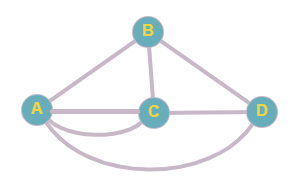# Parks in Exercises 23 and 24, a map of a park is shown with bridges connecting islands in a river to the banks. Represent the map as a graph. See Figures 5.3 and 5.4 on page 234. Is it possible to take a walk that crosses each bridge once and returns to the starting point without crossing any bridge twice? If not, can you do it if you do not end at the starting point? Explain how you know.### Mathematical Excursions (MindTap C...

4th Edition
Richard N. Aufmann + 3 others
Publisher: Cengage Learning
ISBN: 9781305965584

#### Solutions

Chapter
Section### Mathematical Excursions (MindTap C...

4th Edition
Richard N. Aufmann + 3 others
Publisher: Cengage Learning
ISBN: 9781305965584
Chapter 5.1, Problem 24ES
Textbook Problem
12 views

## Parks in Exercises 23 and 24, a map of a park is shown with bridges connecting islands in a river to the banks. Represent the map as a graph. See Figures 5.3 and 5.4 on page 234. Is it possible to take a walk that crosses each bridge once and returns to the starting point without crossing any bridge twice? If not, can you do it if you do not end at the starting point? Explain how you know.To determine

(a)

To graph:

To represent the given map as a graph.

### Explanation of Solution

Given information:

Given map of a park with bridges connecting islands in a river to the banks is

Calculation:

To represent the map as a graph, each land is considered a vertex and each bridge is considered an edge...

To determine

(b)

To determine if it is possible to take a walk that crosses each bridge once and returns to the starting point without crossing any bridge twice. If not, to check if the same is possible without ending at the starting point.

### Still sussing out bartleby?

Check out a sample textbook solution.

See a sample solution

#### The Solution to Your Study Problems

Bartleby provides explanations to thousands of textbook problems written by our experts, many with advanced degrees!

Get Started

Find more solutions based on key concepts
In problems 5-8, Use a calculator to evaluate the indicated powers. 5.

Mathematical Applications for the Management, Life, and Social Sciences

In Exercises 4562, find the values of x that satisfy the inequality (inequalities). 46. 6 4 + 5x

Applied Calculus for the Managerial, Life, and Social Sciences: A Brief Approach

In Exercises 516, evaluate the given quantity. log4116

Finite Mathematics and Applied Calculus (MindTap Course List)

Divide: 48+6

Elementary Technical Mathematics

Classify as true or false. a 54 c 45 b 55 d 55

Elementary Geometry for College Students

The graph of f(x) = 3x5 − 5x3 is:

Study Guide for Stewart's Single Variable Calculus: Early Transcendentals, 8th

The moment about the xz-plane of solid E whose density is ρ(x, y, z) = x is:

Study Guide for Stewart's Multivariable Calculus, 8th

Fill in the blanks. f-gx= __________

College Algebra (MindTap Course List)

In Problems 47–54 find the eigenvalues and eigenvectors of the given matrix. 48.

A First Course in Differential Equations with Modeling Applications (MindTap Course List)

In alphabetical order, the six most common last names in the United States are Brown, Johnson, Jones, Miller,...

Modern Business Statistics with Microsoft Office Excel (with XLSTAT Education Edition Printed Access Card) (MindTap Course List)

Radioactive Decay The half-life of a radioactive substance is the time H that it takes for half of the substanc...

Functions and Change: A Modeling Approach to College Algebra (MindTap Course List)